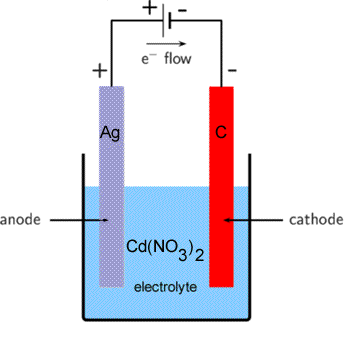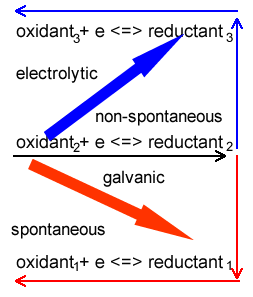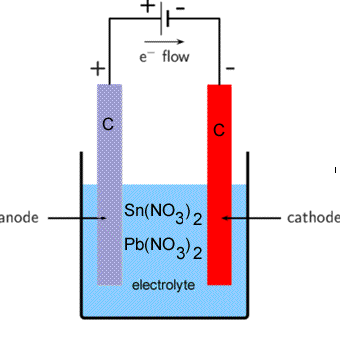Predicting equations and products from electrolysis Consider the electrolytic cell shown on the right. What are the products formed at each electrode?:When predicting products in electrolytic cells it is important to note that in electrolytic cells, unlike galvanic cells, the strongest oxidant reacts with the strongest reductant above it on the electrochemical series. Now to answer the question above take the following steps. Step 1 Identify all the oxidants and reductants present. Keep in mind that water is both an oxidant and a reductant. Oxidants Co2+(aq) , H2O(l) Reductants Ag(s), H2O(l) Step 2 Select the strongest oxidant that will react at the cathode (-) In this case it is Cd2+(aq) and write down the balanced half equation as it appears on the electrochemical series. Cd2+(aq) + 2e => Cd(s) Step 3 Select the strongest reductant that appears above the oxidant. It is this reductant that will most likley react with the oxidant at the anode(+). Write the reaction backwards as it appears on the electrochemical series. Ag(s) => Ag+(aq + eConsider the electrolytic cell shown on the right. The electrolyte contains tin(II)nitrate and lead nitrate. Give the products formed at each electrode and write the balanced half equations of the reactions that take place at each electrode. Step 1 Identify all the oxidants and reductants present. Keep in mind that water is both an oxidant and a reductant. Oxidants Sn2+(aq) ,Pb2+(aq), H2O(l) Reductants Sn2+(aq), H2O(l) Step 2 Select the strongest oxidant that will react at the cathode (-) In this case it is Pb2+(aq), write down the balanced half equation as it appears on the electrochemical series. Pb2+(aq) + 2e => Pb(s) Step 3 Select the strongest reductant, that appears above the oxidant, in this case it is Sn2+(aq). Now write the reaction backwards as it appears on the electrochemical series. Sn2+(aq) => Sn4+(aq) + e Animated solutionFor each of the following give the products formed at the anode and the cathode and write the balanced half equations for each reaction that occurs at the anode and cathode. 1) An electrolytic cell is composed of a molten MgCl2 and two carbon electrodes. solution 2) An electrolytic cell is composed of a 0.1M CuSO4 and two carbon electrodes. solution 3) An electrolytic cell is composed of a molten CaCl2 and two carbon electrodes. Solution